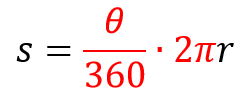# Arc Length (s) versus Radius (r) - Constant of Proportionality

Author:
kathleenh

Find the arc length (s) when and , , , , and .Below is the s vs. r graph (arc length vs. radius) for radius values of 1, 2, 3, 4 and 5.

## Plotting s vs r for r=1, 2, 3, 4, 5 gives the following graph:

Consider the formula for arc length (s) in terms of radius (r).Plotting s vs r with for every angle gives a linear relationship. What is the slope of this line?

Check all that apply

## Adjust the angle slider. How does the slope of the line change for different angle measures? At higher angle measures, zoom out to see all the plotted points.

The arc length (s) and the radius (r) are directly proportional, meaning they increase and decrease at the same rate. The ratio between two directly proportional quantities is called the constant of proportionality. The constant of proportionality for s / r is the slope of the line (graphed above) or . This is how the radian measure for an angle is defined, where . Simplifying this gives a formula for finding the radian measure of any angle. Which is the correct formula?

Check all that apply

Use the formula for radians, , to select all of the correct radian measures below.

Check all that apply